### Constructions Class 10th Mathematics Gujarat Board Solution

##### Question 1.Draw a circle of radius 5 cm. From a point 8 cm away from the centre, construct two tangents to the circle from this point. Measure them.Answer:Steps of Construction:1. Draw a circle of radius 5cm and center O.2. Draw a line OP such that OP= 8cm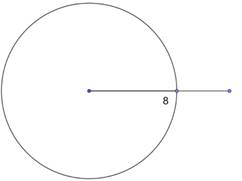3. Draw a perpendicular bisector of OP which cuts OP at M.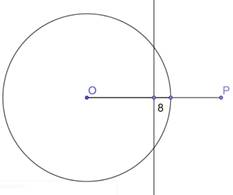4. Taking M as center and radius OM, draw a circle.5. The two circles intersect at A and B.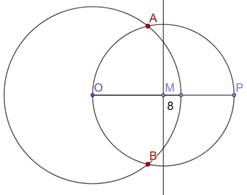6. Join AP and BP. AB and BP are the required tangents.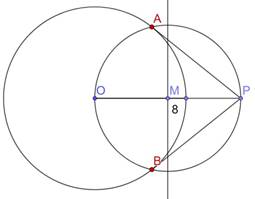Question 2.Draw ⨀ (O, 4). Construct a pair of tangents from A where OA = 10 units.Answer:Steps of Construction:1. Draw a circle of radius 4cm and center O.2. Draw a line OA such that OA= 10cm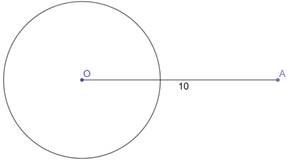3. Draw a perpendicular bisector of OA which cuts OA at M.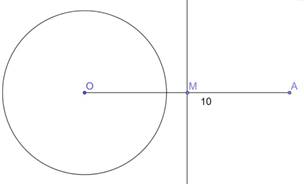4. Taking M as center and radius OM, draw a circle.5. The two circles intersect at P and Q.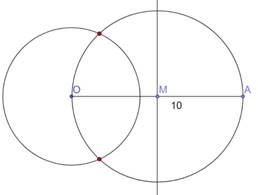6. Join AP and AQ. AP and AQ are the required tangents.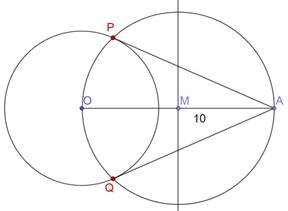Question 3.Draw a circle with the help of a circular bangle. Construct two tangents to this circle through a point in the exterior of the circle.Answer:Steps of construction:1. Draw a circle(Say C1) with the help of a bangle, and take any three points A, B and C on its circumference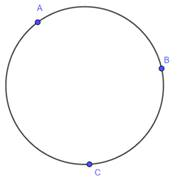2. Join AB and BC and draw the perpendicular bisector of AB and BC which intersect each other at O.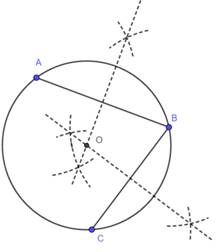3. O is the center of circle, now take any point P outside the circle and join OP.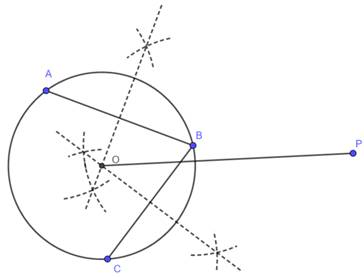4. Draw the perpendicular bisector of OP, which intersect OP at O'.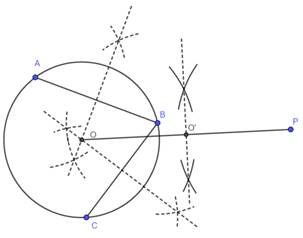5. Taking O' as center and O'O = O'P as radius, draw a circle C2, which intersects the previous circle at Q and R.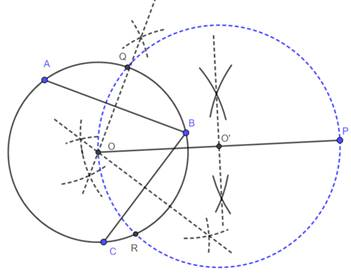6. Join PQ and PR, which are required tangents.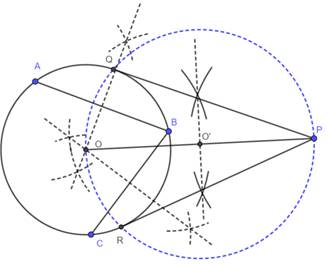Question 4.Draw ⨀(O, r).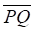is a diameter of 0(0, r) . Points A and B are on the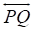such that A—P—Q and P—Q—B. Construct tangents through A and B to ⨀(O, r) .Answer:Steps of Construction:1. Draw a circle of any radius(r ) and center O. Draw a diameter PQ of this circle.2. Extend PQ both the sides as APQB is a straight line.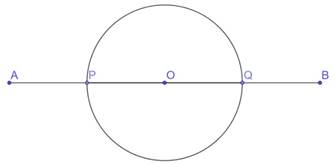3. Draw a perpendicular bisector of OA and OB which cuts OA and OB.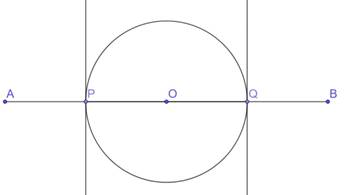4. Taking OA and OB as diameters, draw two circles.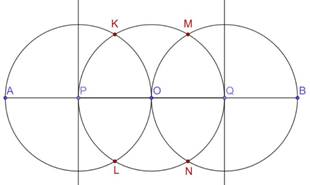5. The three circles intersect at K,L and M,N.6. Join AK and AL. Join BM and BN.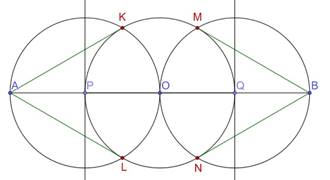7. AK,AL,BM and BN are the required tangents.Question 5.Draw AB such that AB = 10 cm. Draw ⨀(A, 3) and ⨀(B, 4). Construct tangents to each circle through the centre of the other circle.Answer:Steps of Construction:1. Draw a circle of any radius 3 and center A.2. With A as center, draw a circle of radius 3 cm. With B as center, draw a circle of radius 4cm.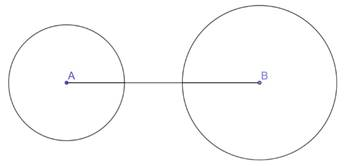3. Draw a perpendicular bisector of AB which cuts AB at M.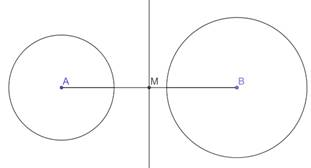4. With M as center and AM as radius, draw a circle.5. The three circles intersect at K,L and M,N.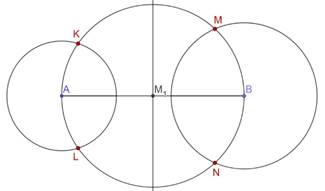6. Join AM and AN. Join BK and BL.7. AM,AN,BK and BL are the required tangents.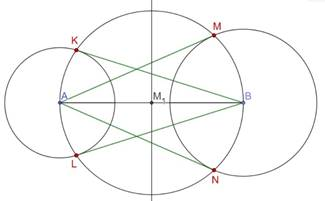Question 6.⨀ (P, 4) is given. Draw a pair of tangents through A which is in the exterior of ⨀(P, 4) such that measure of an angle between the tangents is 60° .Answer:Angle between the tangents =60°We first find the angle between the radius.Angle between radius + Angle between tangents =180°⇒ Angle between radius = 180-60=120°Steps of Construction:1. Draw a circle of radius 4cm and center P.2. Draw a radius on the circle. Draw another radius making 120° with this radius.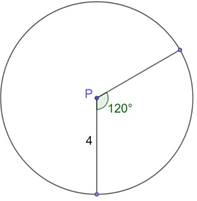3. From the points on the circumference on the circle, draw perpendiculars to these radius.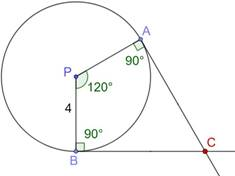4. They intersect each other at one point.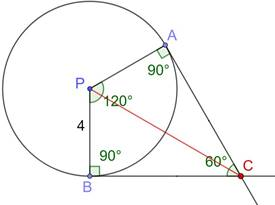PDF FILE TO YOUR EMAIL IMMEDIATELY PURCHASE NOTES & PAPER SOLUTION. @ Rs. 50/- each (GST extra)

HINDI ENTIRE PAPER SOLUTION

MARATHI PAPER SOLUTION

SSC MATHS I PAPER SOLUTION

SSC MATHS II PAPER SOLUTION

SSC SCIENCE I PAPER SOLUTION

SSC SCIENCE II PAPER SOLUTION

SSC ENGLISH PAPER SOLUTION

SSC & HSC ENGLISH WRITING SKILL

HSC ACCOUNTS NOTES

HSC OCM NOTES

HSC ECONOMICS NOTES

HSC SECRETARIAL PRACTICE NOTES

# 2019 Board Paper Solution

HSC ENGLISH SET A 2019 21st February, 2019

HSC ENGLISH SET B 2019 21st February, 2019

HSC ENGLISH SET C 2019 21st February, 2019

HSC ENGLISH SET D 2019 21st February, 2019

SECRETARIAL PRACTICE (S.P) 2019 25th February, 2019

HSC XII PHYSICS 2019 25th February, 2019

CHEMISTRY XII HSC SOLUTION 27th, February, 2019

OCM PAPER SOLUTION 2019 27th, February, 2019

HSC MATHS PAPER SOLUTION COMMERCE, 2nd March, 2019

HSC MATHS PAPER SOLUTION SCIENCE 2nd, March, 2019

SSC ENGLISH STD 10 5TH MARCH, 2019.

HSC XII ACCOUNTS 2019 6th March, 2019

HSC XII BIOLOGY 2019 6TH March, 2019

HSC XII ECONOMICS 9Th March 2019

SSC Maths I March 2019 Solution 10th Standard11th, March, 2019

SSC MATHS II MARCH 2019 SOLUTION 10TH STD.13th March, 2019

SSC SCIENCE I MARCH 2019 SOLUTION 10TH STD. 15th March, 2019.

SSC SCIENCE II MARCH 2019 SOLUTION 10TH STD. 18th March, 2019.

SSC SOCIAL SCIENCE I MARCH 2019 SOLUTION20th March, 2019

SSC SOCIAL SCIENCE II MARCH 2019 SOLUTION, 22nd March, 2019

XII CBSE - BOARD - MARCH - 2019 ENGLISH - QP + SOLUTIONS, 2nd March, 2019

# HSCMaharashtraBoardPapers2020

(Std 12th English Medium)

HSC ECONOMICS MARCH 2020

HSC OCM MARCH 2020

HSC ACCOUNTS MARCH 2020

HSC S.P. MARCH 2020

HSC ENGLISH MARCH 2020

HSC HINDI MARCH 2020

HSC MARATHI MARCH 2020

HSC MATHS MARCH 2020

# SSCMaharashtraBoardPapers2020

(Std 10th English Medium)

English MARCH 2020

HindI MARCH 2020

Hindi (Composite) MARCH 2020

Marathi MARCH 2020

Mathematics (Paper 1) MARCH 2020

Mathematics (Paper 2) MARCH 2020

Sanskrit MARCH 2020

Sanskrit (Composite) MARCH 2020

Science (Paper 1) MARCH 2020

Science (Paper 2)

Geography Model Set 1 2020-2021

MUST REMEMBER THINGS on the day of Exam

Are you prepared? for English Grammar in Board Exam.

Paper Presentation In Board Exam

How to Score Good Marks in SSC Board Exams

Tips To Score More Than 90% Marks In 12th Board Exam

How to write English exams?

How to prepare for board exam when less time is left

How to memorise what you learn for board exam

No. 1 Simple Hack, you can try out, in preparing for Board Exam

How to Study for CBSE Class 10 Board Exams Subject Wise Tips?

JEE Main 2020 Registration Process – Exam Pattern & Important Dates

NEET UG 2020 Registration Process Exam Pattern & Important Dates

How can One Prepare for two Competitive Exams at the same time?

8 Proven Tips to Handle Anxiety before Exams!